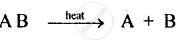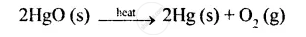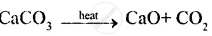ICSE Class 8CISCE
Share

# Explain the Following Types of Chemical Reactions Giving Two Examples for Each of Them Decomposition Reaction . - ICSE Class 8 - Chemistry

ConceptTypes of Reactions - Decomposition

#### Question

Explain the following types of chemical reactions giving two examples for each of them decomposition reaction .

#### Solution

Decomposition reaction: A reaction in which a compound breaks up due to the application of heat into two or more simple substances is called decomposition reaction.e.g. (i) Mercuric oxide when heated, decomposes to form two elements mercury and oxygen(ii) CaCO3 when heated decomposes to calcium oxide and carbon dioxide.Is there an error in this question or solution?
Solution Explain the Following Types of Chemical Reactions Giving Two Examples for Each of Them Decomposition Reaction . Concept: Types of Reactions - Decomposition.
S